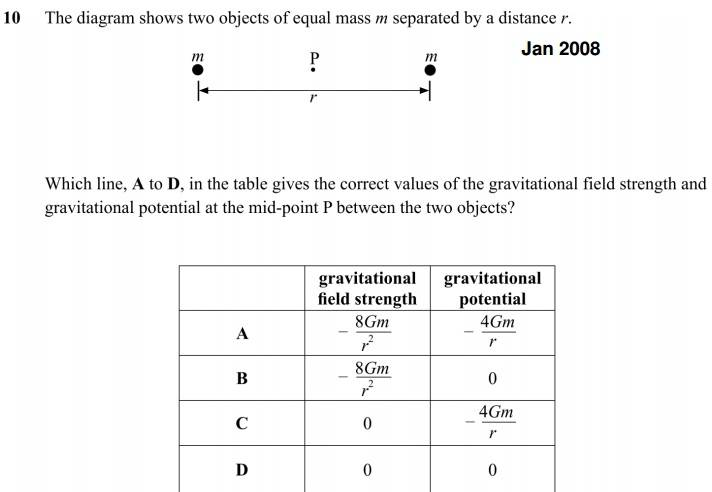# Gravitational potential

## Homework Statement## Homework Equations

g = G*M / r^2, where g is the gravitational field strength, G is the gravitational constant, M is the mass of the attracting body, r is the distance from the center of mass of the body.
V = -G*M / r, where V is the gravitational potential.

## The Attempt at a Solution

For the gravitational field strength, as P is at a midpoint, we can say that r is 1/2*r, and as the masses are also equal, G*M / 1/2*r^2 = G*M / 1/2*r^2 (as they are equal, and in opposite directions), and they will simply cancel to 0. Therefore, it can't be A or B.

For gravitational potential, again, the masses are equal, and r is 1/2*r, so -GM / 1/2*r = -GM / 1/2*r, they will again cancel to 0. However, the answer is apparently C, where gravitational potential is -4G*M / r? I can't see why.

## Answers and Replies

BvU
Science Advisor
Homework Helper
In V = -G*M / r there are no vectors, only scalars. r is a distance in this formula.

(dividing by a vector is awkward....)

In V = -G*M / r there are no vectors, only scalars. r is a distance in this formula.

(dividing by a vector is awkward....)
I see, so then you're left with -2GM / r + -2GM / r, my mistake.

mfb
Mentor
The problem statement should clarify that the potential "at infinity" is defined as zero. Otherwise the question is ambiguous - you can always add a constant value to the potential without changing physics.
Using this, you can rule out answer (D) simply from the fact that an object there would need energy to escape, therefore the potential there cannot be zero.